Courses

# Water Pollution Control by Adsorption (Part - 1) Computer Science Engineering (CSE) Notes | EduRev

## Computer Science Engineering (CSE) : Water Pollution Control by Adsorption (Part - 1) Computer Science Engineering (CSE) Notes | EduRev

The document Water Pollution Control by Adsorption (Part - 1) Computer Science Engineering (CSE) Notes | EduRev is a part of the Computer Science Engineering (CSE) Course Environmental Engineering - Notes, Videos, MCQs & PPTs.
All you need of Computer Science Engineering (CSE) at this link: Computer Science Engineering (CSE)

• Adsorption can be simply defined as the concentration of a solute, which may be molecules in a gas stream or a dissolved or suspended substance in a liquid stream, on the surface of a solid.
• In an adsorption process, molecules or atoms or ions in a gas or liquid diffuse to the surface of a solid, where they bond with the solid surface or are held there by weak intermolecular forces. The adsorbed solute is called the adsorbate, and the solid material is the adsorbent.
• Activated clays, activated carbons, fuller earths, bauxite, alumina, bone char, molecular sieves, synthetic polymeric adsorbents, silica gel, etc. are the main types of adsorbents used in the industry.
• There are basically two types of adsorption processes: one is physical adsorption (physisorption) and the second is chemisorption.

There are essentially four stages in the adsorption of an organic/inorganic species by a porous adsorbent:

1. Transport of adsorbate from the bulk of the solution to the exterior film surrounding the adsorbent particle;

2. Movement of adsorbate across the external liquid film to the external surface sites on the adsorbent particle (film diffusion);

3. Migration of adsorbate within the pores of the adsorbent by intraparticle diffusion (pore diffusion);

All these processes play a role in the overall sorption within the pores of the adsorbent. In a rapidly stirred, well mixed batch adsorption, mass transport from the bulk solution to the external surface of the adsorbent is usually fast. Therefore, the resistance for the transport of the adsorbate from the bulk of the solution to the exterior film surrounding the adsorbent may be small and can be neglected. In addition, the adsorption of adsorbate at surface sites (step 4) is usually very rapid and thus offering negligible resistance in comparison to other steps, i.e. steps 2 and 3. Thus, these processes usually are not considered to be the rate-limiting steps in the  sorption process.

In most cases, steps (2) and (3) may control the sorption phenomena.

For the remaining two steps in the overall adsorbate transport, three distinct cases may occur:

Case I:  external transport > internal transport.

Case II:  external transport < internal transport.

Case III:  external transport ≈ internal transp ort.

In cases I and II, the rate is governed by film and pore diffusion, respectively. In case III, the transport of ions to the boundary may not be possible at a significant rate, thereby, leading to the formation of a liquid film with a concentration gradient surrounding the adsorbent particles.

Usually, external transport is the rate-limiting step in systems which have (a) poor phase mixing, (b) dilute concentration of adsorbate, (c) small particle size, and (d) high affinity of the adsorbate for the adsorbent. In contrast, the intra-particle step limits the overall transfer for those systems that have (a) a high concentration of adsorbate, (b) a good phase mixing, (c) large particle size of the adsorbents, and (d) low affinity of the adsorbate for adsorbent . The possibility of intra-particle diffusion can be explored using the intra-particle diffusion model .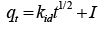(3.12.1)
Where, qt is th e amount of the adsorbate adsorbed on the adsorbent (mg/g) at any t and  is the intra-particle diffusion rate constant, and values of I give an idea about the thickness of the boundary layer.  In order to check whether surface diffusion controls the adsorption process, the kinetic data can be analyzed using Boyd kinetic expression which is given by: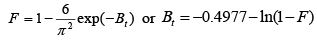(3.12.2)
Where, F(t) = q/qe is the fractional attainment of equilibrium at time t, and Bt is a mathematical function of F.   However, if the data exhibit multi-linear plots, then two or more steps influence the overall adsorption process. In general, external mass transfer is characterized by the initial solute uptake and can be calculated from the slope of plot between C/Co versus time. The slope of these plots can be calculated either by assuming polynomial relation between C/Co and time or it can be calculated based on the assumption that the relationship was linear for the first initial rapid phase.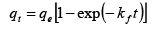(3.12.3 )
where, qe is the amount of the adsorbate adsorbed on the adsorbent under equilibrium condition, kf is the pseudo-first order rate constant.   The pseudo-second-order model is represented as :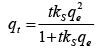(3.12.4)
The initial sorption rate, h (mg/g min), at t -> 0 is defined as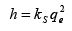(3.12.5)

Equilibrium adsorption equations are required in the design of an adsorption system and their subsequent optimization . Therefore it is important to establish the most appropriate correlation for the equilibrium isotherm curves  have discussed the theory associated with the most commonly used isotherm models. Various isotherms namely Freundlich, Langmuir, Redlich-Peterson (R-P) and Tempkin which are given in following table are widely used to fit the experimental data:

Table 3.12.2. Various isotherm equations for the adsorption process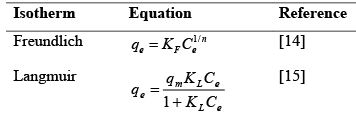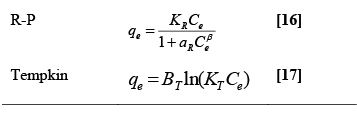KR: R–P isotherm constant (l/g), aR: R–P isotherm constant (l/mg), β: Exponent which lies between 0 and 1, Ce: Equilibrium liquid phase concentration (mg/l), KF: Freundlich constant (l/mg), 1/n: Heterogeneity factor, KL: Langmuir adsorption constant (l/mg), qm: adsorption capacity (mg/g), KT: Equilibrium binding constant (l/mol), BT: Heat of adsorption.

The Freundlich isotherm is derived by assuming a heterogeneous surface with a nonuniform distribution of heat of adsorption over the surface, whereas in the Langmuir theory the basic assumption is that the sorption takes place at specific homogeneous sites within the adsorbent. The R-P isotherm incorporates three parameters and can be applied either in homogenous or heterogeneous systems. Tempkin isotherm assumes that the heat of adsorption of all the molecules in the layer decreases linearly with coverage due to adsorbent-adsorbate interactions, and the adsorption is characterized by a uniform distribution of binding energies, up to some maximum binding energy.

Offer running on EduRev: Apply code STAYHOME200 to get INR 200 off on our premium plan EduRev Infinity!

,

,

,

,

,

,

,

,

,

,

,

,

,

,

,

,

,

,

,

,

,

;